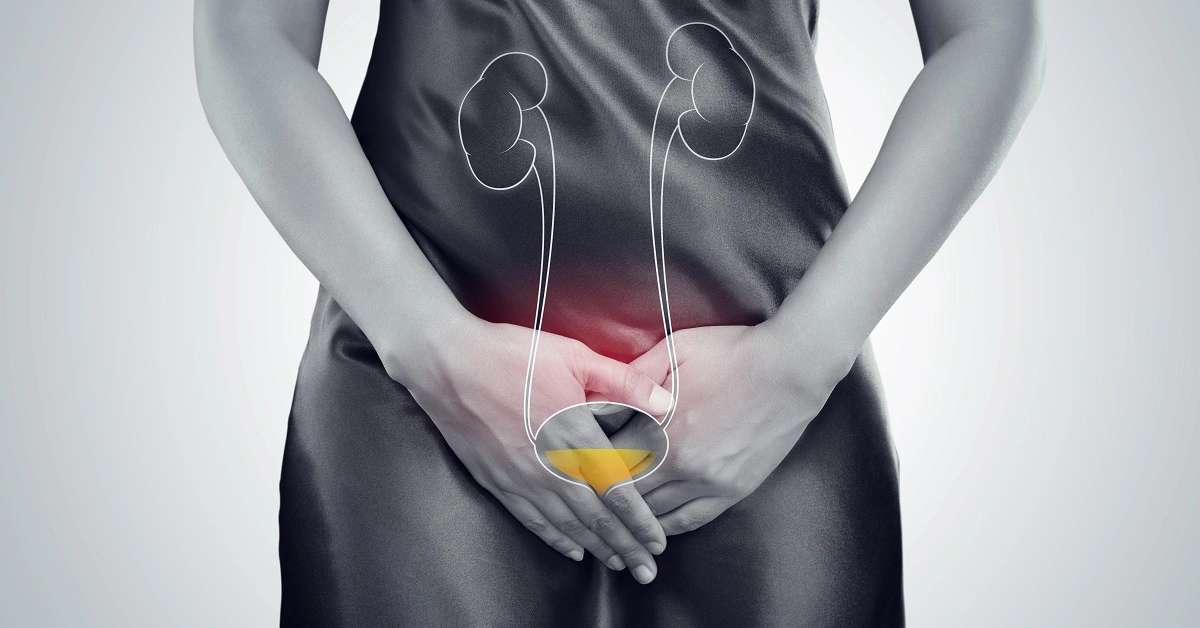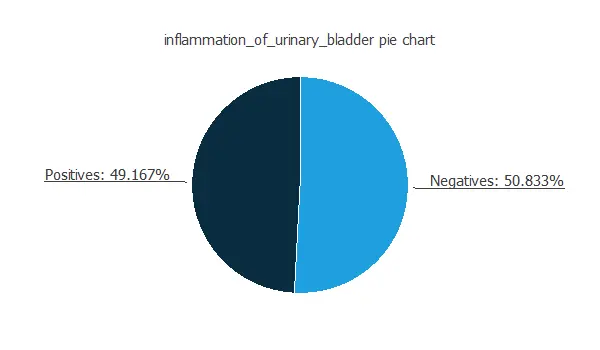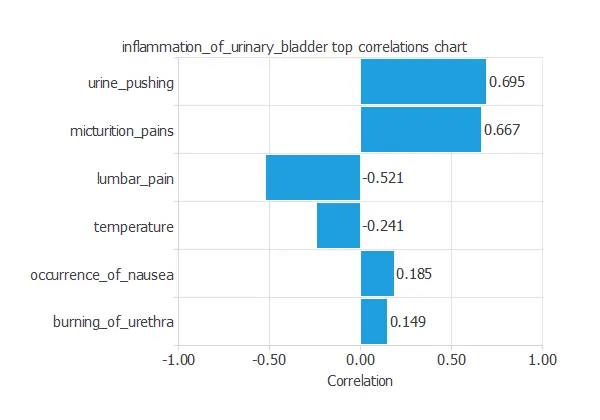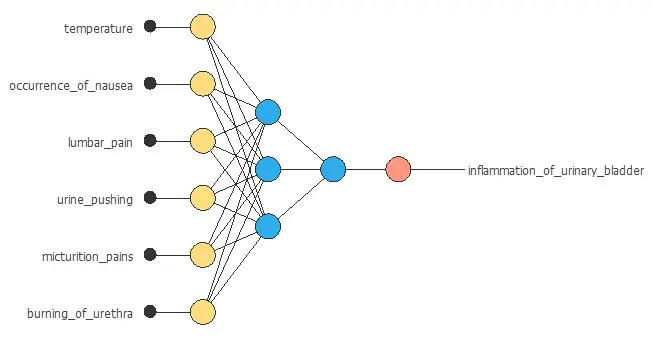# Diagnose urinary inflammations using artificial intelligence

This example aims to design a model that can diagnose acute inflammation or nephritises of the urinary bladder using machine learning.

This is a medical diagnosis application.

The main idea of this algorithm is to perform a presumptive diagnosis of two diseases of the urinary system. Afterward, an expert will make a confirmatory diagnosis to verify the results.

With proper treatment, symptoms of inflammation usually decay within a few days. However, there is an inclination to relapse. Therefore, in people with acute urinary bladder inflammation, we should expect that the illness will turn into a long-form.

For this, we used a dataset created by a medical expert for the presumptive diagnosis of two diseases of the urinary system. See references.### Contents:

This example is solved with Neural Designer. To follow it step by step, you can use the free trial.

## 1. Application type

This is a classification project. Indeed, we want to predict binary variables.

The goal here is to model the probability of nephritises and urinary bladder inflammation conditioned on the patient's symptoms.

Since both variables are binary and independent, we will use the model to predict the diseases one at a time. In this example, Neural Designer will build the model to predict acute inflammation of the urinary bladder.

## 2. Data set

The data file urinary_inflammation.csv contains the data for this example. The number of instances (rows) is 120, and the number of variables (columns) is 8.

The number of input variables, or attributes for each sample, is 8. All input variables, except temperature, are binary and represent the patients' symptomatology (pain, nausea, burning sensation, etc.). This data set contains the following variables summarizzed as a list:

• temperature, in Celsius degrees, body temperature of the patient.
• nausea: (1-0), if the patient feels nauseated while urinating.
• lumbar_pain: (1-0), if the patient has lumbar pain while urinating.
• urine_pushing: (1-0), urgent need to urinate even when the bladder is empty.
• micturition_pain: (1-0), if the patient feels pain while urinating.
• burning_of_urethra: (1-0), wether or not the patient has a burning sensation while urinating.
• nephritis_of_renal_pelvis_origin: (1-0), used as the target.
• inflammation_of_urinary_bladder: (1-0), used as the target.

The goal is to get a model that can diagnose one of the diseases. Therefore, we set nephritis_of_renal_pelvis_origin will as unused, and inflammation_of_urinary_bladder as target.

The instances are divided into training, selection, and testing subsets, and set for use. Neural Designer automatically assigns the instances to 60% (72) for training, 20% (24) for generalization, and 20% (24) for testing of the original instances, and are split at random. The user can choose to modify these values.

Before the model configuration, we recommend performing an exploratory analysis of the data we are using. For example, classification projects must know the distribution of the target variable in the dataset. The following picture shows a pie chart for the inflammation_of_urinary_bladder variable.As we can see, we have the same percentage of each sample type. Therefore, the data is balanced. We will later use this information to define the parameters of the neural network.

Another relevant piece of information to keep in mind is the correlation of each input with the target variable. The chart below displays this information.From the picture above, we can conclude that the variables with a considerable influence on the target variable are urine pushing and micturition pain, and lumbar pain.

## 3. Neural network

The next step is to choose a neural network to represent the classification function. For classification problems, the neural network is composed of:

The scaling layer contains the statistics of the inputs calculated from the input data and the method for scaling the input variables. In this case we will use the mean and standard deviation scaling method for the temperature variable, as it is a continuous variable, and the minimum-maximum method for all the other variables, because they are binary.

A perceptron layer with a hidden logistic layer. The neural network must have six inputs since the number of scaling neurons is six. As an initial guess, we use three neurons in the hidden layer.

Finally, we will set the binary probabilistic method for the probabilistic layer, as we want the predicted target variable to be binary. We can interpret this output as a probability of class membership. This layer has three inputs but only has one output. This value is the probability of the sample corresponding to a patient having bladder inflammation.

The following figure is a graphical representation of the neural network described above.## 4. Training strategy

The fourth step is to set the training strategy, which is composed of:

• A loss index.
• An optimization algorithm.

The loss index is the weighted squared error with L2 regularization. This is the default loss index for binary classification applications.

The optimization algorithm that we use is the quasi-Newton method. This is also the standard optimization algorithm for this type of problem.

We can state the learning problem as finding a neural network that minimizes the loss index. That is, a neural network that fits the data set (error term) and does not oscillate (regularization term).

The optimization algorithm set for the model is the quasi-Newton method. This is also the standard optimization algorithm for this type of problem.

The following chart shows how the error decreases with the iterations during the training process. The final training and selection errors are training error = 0.007 WSE and selection error = 0.005 WSE, respectively.## 5. Model selection

The objective of model selection is to improve the generalization capabilities of the neural network or, in other words, to reduce the selection error on the selected instances of the data set.

Since the selection error that we have achieved so far is minimal 0.005, we don't need to apply order selection nor input selection here.

## 6. Testing analysis

An exhaustive testing analysis is performed to validate the generalization performance of the trained neural network. To validate a classification technique, we need to compare the values provided by this technique to the observed values.We can use the ROC curve as it is the standard testing method for binary classification projects.A random classifier has an area under a curve of 0.5, while a perfect classifier has an area under the curve AUC = 1. In practice, this measure should take a value between 0.5 and 1. The closer to 1, the better the classifier. In this example, this parameter is AUC = 1.

The following table contains the elements of the confusion matrix. The variable diagnosis contains the true positives, false positives, true negatives, and false negatives.

Predicted positive Predicted negative
Real positive 15 0
Real negative 0 9

The number of correctly classified instances is 24, and the number of misclassified instances is 0. From this table, we can calculate the binary classification tests.

The binary classification tests are parameters for measuring the performance of a classification problem with two classes:

• Classification accuracy (ratio of instances correctly classified): 100%
• Error rate (ratio of instances misclassified): 0%
• Sensitivity (ratio of real positive which are predicted positive): 100%
• Specificity (ratio of real negative which are predicted negative): 100%

From the results above, we can say that the model is predicting perfectly.

## 7. Model deployment

The neural network is now ready to predict outputs for inputs that it has never seen.

We calculate the neural network outputs to diagnose inflammation of urinary bladder from the characteristics of a new patient. The following list shows some values for the inputs.

• temperature: 38.7.
• nausea: 1 (yes).
• lumbar_pain: 1 (yes).
• urine_pushing: 0 (no).
• micturition_pain: 0 (no).
• burning_of_urethra: 1 (yes).

For these inputs, the predicted values are the following:

• nephritis_of_renal_pelvis_origin: 5.14%.

We can also use Response Optimization. The objective of the response optimization algorithm is to exploit the mathematical model to look for optimal operating conditions.
Indeed, the predictive model allows us to simulate different operating scenarios and adjust the control variables to improve efficiency.

An example is to minimize the urinary bladder inflammation probability while maintaining the value of urethra burning equal to 1.

The next table resumes the conditions for this problem.

Variable name Condition
Temperature None
Nausea None
Urine pushing None
Micturition pain None
Burning of urehtra Equal to 1
Nephritis of renal pelvis origin None

The next list shows the optimum values for previous conditions.

• temperature: 37.04.
• nausea: 1 (yes).
• lumbar_pain: 0 (no).
• urine_pushing: 0 (no).
• micturition_pain: 0 (no).
• burning_of_urethra: 1 (yes).
• nephritis_of_renal_pelvis_origin: 99.66%.

Furthermore, we can export the mathematical expression of the neural network to a dedicated diagnosis software used by doctors. The expression is listed below.

```scaled_temperature = (temperature-38.7242012)/1.819129944;
scaled_occurrence_of_nausea = occurrence_of_nausea*(1+1)/(1-(0))-0*(1+1)/(1-0)-1;
scaled_lumbar_pain = lumbar_pain*(1+1)/(1-(0))-0*(1+1)/(1-0)-1;
scaled_urine_pushing = urine_pushing*(1+1)/(1-(0))-0*(1+1)/(1-0)-1;
scaled_micturition_pains = micturition_pains*(1+1)/(1-(0))-0*(1+1)/(1-0)-1;
scaled_burning_of_urethra = burning_of_urethra*(1+1)/(1-(0))-0*(1+1)/(1-0)-1;

perceptron_layer_1_output_0 = logistic( -0.124535 + (scaled_temperature*-0.280739) + (scaled_occurrence_of_nausea*-0.723146) + (scaled_lumbar_pain*-1.19733) + (scaled_urine_pushing*-1.51965) + (scaled_micturition_pains*-0.81476) + (scaled_burning_of_urethra*0.134913) );
perceptron_layer_1_output_1 = logistic( -0.12515 + (scaled_temperature*-0.280009) + (scaled_occurrence_of_nausea*-0.723488) + (scaled_lumbar_pain*-1.19886) + (scaled_urine_pushing*-1.52104) + (scaled_micturition_pains*-0.815837) + (scaled_burning_of_urethra*0.135458) );
perceptron_layer_1_output_2 = logistic( 0.1408 + (scaled_temperature*0.296888) + (scaled_occurrence_of_nausea*0.849916) + (scaled_lumbar_pain*1.40837) + (scaled_urine_pushing*1.77715) + (scaled_micturition_pains*0.932039) + (scaled_burning_of_urethra*-0.155899) );

probabilistic_layer_combinations_0 = 0.684833 -2.57991*perceptron_layer_1_output_0 -2.58307*perceptron_layer_1_output_1 +3.56417*perceptron_layer_1_output_2